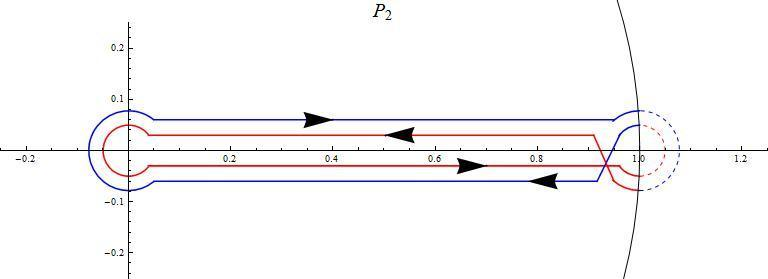# Which algebraic branches does a pochammer contour traverse?

Which algebraic branches does a pochhammer contour traverse?

Hi,

Got another question about pochhammer continuation of beta function in regards to algebraic function cycles:

Which cycles of a function does the pochhammer contour traverse? Take for example the function:

$$w=z^{4/5}(1-z)^{3/7}$$

That corresponds to the expresson:

$$f(z,w)=z^{28}(1-z)^{15}-w^{35}=0$$

We have 35 coverings ramifying at the origin into seven 5-cycle branches. Suppose I pin one of the horizontal legs of the pochhammer contour over one determination of one of those seven branches. Which determination and which branches will the remainder of the contour traverse? Can this be predicted for any algebraic function? Will it remain on only one branch or will it traverse two three or four different branches? I don't know.

I suppose I could expand the function into it's Puiseux series, pin the contour at a reference point, say ##z=1/10##, track it around, selecting the four values at the reference point, compare those values with each series at the reference point, and compare the results. That is however a completely empirical and brute-force approach and I was wondering if there is a more algebraic way of doing this.

Ok thanks,
Jack

Last edited:

I've made some progress with this matter:

(1) If the function is fully-ramified at the origin, then obviously the contour remains on the same branch. This is the case for functions having the form:

$$w=z^{1/d}(1-z)$$

which is equivalent to

$$f(z,w)=z(1-z)^d-w^d=0$$

which can be easily shown to be fully-ramified at the origin.

(2) The maximum number of branches the contour can traverse is only two and not four as I alluded to above. For one thing, on the return trip, the contour must return to the starting determination since the path is closed over the function. This can be easily shown in the following figure. The solid blue and red paths are analytically continuous over the same branch but they may be different branches.So the question now becomes: if the function has multiple branches about the origin, when will the pochhammer contour remain on the same branch, if it ever does, and if it traverses two, which two?

Let's take a simple case to study experimentally:

$$w=z^{1/2}(1-z)^{1/3}$$

which has three 2-cycle branches about the origin. So the problem becomes:

$$w_{2,1}\to ?$$
$$w_{2,2}\to ?$$
$$w_{2,3}\to ?$$

where the subscripts order the branches in some selected order. So, starting the contour over each branch, does it stay on the branch and if not, which branch does it go to?

#### Attachments

Last edited by a moderator:
So, starting the contour over each branch, does it stay on the branch and if not, which branch does it go to?

I wish to argue that the contour never stays on the same branch for this function without doing any sort of experimental testing:

Since the branch is 2-cycle and the contour traverses only two determinations, then in order for the contour to remain on the branch, it would have to traverse all determinations. In order for the contour to do this, the branching geometry outside the unit circle must support 2-cycle branching. However, since z=1 is the only remaining singular point, that branching geometry is precisely the geometry around the point at infinity and it is a simple matter to show the function fully-ramifies there. Therefore, it is impossible for the contour to remain on the same branch so must traverse a second one.

Last edited: